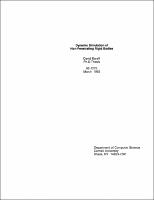## Dynamic Simulation of Non-Penetrating Rigid Bodies##### Abstract
This thesis examines the problems and difficulties in the forward dynamic simulation of rigid bodies subject to non-penetration constraints. By adopting a simple but well-defined model of rigid body dynamics, we are able to focus on and gain insight into some of the inherent difficulties of rigid body simulation. Additionally, computationally practical solutions to some of the problems encountered in this thesis are presented. Enforcing non-penetration constraints is essentially a two step process. The first step of the simulation involves the detection of potential contacts between bodies. This thesis presents collision detection algorithms for the dynamic simulation of bodies that are composed of both polyhedra and convex closed curved surfaces. The collision detection algorithms exploit temporal coherence to achieve fast running times and are a practical solution to the problem of collision detection during simulation. The second step of the simulation involves the computation of the contact forces between bodies that maintain the non-penetration constraint. This thesis considers first the problem of computing contact forces between a pair of bodies that contact at a point without friction. A mathematical formulation for the contact force between the bodies is presented, and then modified to yield a formulation that is computationally practical for use in a simulator. After considering the dynamics of single point contacts, systems with multiple contacts are considered both in terms of computational complexity measures and practical solution methods. The methods used in this thesis to compute constraint forces are also theoretically and practically compared with a popular method for preventing inter-penetration called the "penalty method". After considering frictionless systems, this thesis considers systems of bodies that behave according to the classical Coulomb model of friction (which includes both sliding and dry friction). This leads us to consider systems in which there are no solutions to the classical constraint force equations, as well as systems which admit multiple solutions for the constraint force equations and whose subsequent behavior is thus indeterminate. Both computational and practical complexity results for simulating such systems are discussed.
1992-03
##### Publisher
Cornell University
##### Keywords
computer science; technical report
##### Previously Published As
http://techreports.library.cornell.edu:8081/Dienst/UI/1.0/Display/cul.cs/TR92-1275
technical report